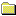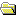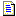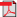Products Download Events Support Videos All Product Families ARM7, ARM9, and Cortex-M3 Products C16x, XC16x, and ST10 Products C251 and 80C251 Products Cx51 and 8051 Products Modified Anytime In the Last 7 Days In the Last 14 Days In the Last 30 Days In the Last 90 Days In the Last 6 Months

Technical Support

On-Line Manuals

Libraries and Floating Point Support GuidePrefaceThe ARM C and C++ LibrariesThe ARM C Micro-libraryFloating-point SupportAbout floating-point supportThe software floating-point library, fplibCalling fplib routinesfplib arithmetic on numbers in a particular formatfplib conversions between floats, long longs, doubfplib comparisons between floats and doublesfplib C99 functionsControlling the ARM floating-point environmentFloating-point functions for compatibility with MiC99-compatible functions for controlling the ARM fC99 rounding mode and floating-point exception macException flag handlingFunctions for handling rounding modesFunctions for saving and restoring the whole floatFunctions for temporarily disabling exceptionsARM floating-point compiler extensions to the C99Writing a custom exception trap handlerExample of a custom exception handlerException trap handling by signalsmathlib double and single-precision floating-pointIEEE 754 arithmeticBasic data types for IEEE 754 arithmeticSingle precision data type for IEEE 754 arithmeticDouble precision data type for IEEE 754 arithmeticSample single precision floating-point values forSample double precision floating-point values forIEEE 754 arithmetic and roundingExceptions arising from IEEE 754 floating-point arException types recognized by the ARM floating-poiUsing the Vector Floating-Point (VFP) support librThe C and C++ Library Functions referenceFloating-point Support Functions Reference

# <!-- var kmNextPage = "armlib_chr1358938945873.htm"; var kmPrevPage = "armlib_chr1358938945358.htm"; var kmNextPageTitle = "Example of a custom exception handler"; var kmPrevPageTitle = "ARM floating-point compiler extensions to the C99"; var kmBreadCrumbs = "<a href=\"default.htm\">Home</a> &raquo; <a href=\"armlib_chr1358938940724.htm\">Floating-point Support</a> &raquo; <a href=\"armlib_chr1358938943345.htm\">Controlling the ARM floating-point environment</a> &raquo; Writing a custom exception trap handler"; kmSetupPaging (); kmNavButtons(); //--> Writing a custom exception trap handler

### 3.3.9 Writing a custom exception trap handler

Custom exception trap handlers let you override the default exception handling behavior. For example, when converting Fortran code you might want to override the division by zero exception to return 1 rather than an invalid operation exception.

### Note

The following functionality requires you to select a floating-point model that supports exceptions, such as `--fpmode=ieee_full` or `--fpmode=ieee_fixed`.
If you want to install a custom exception trap handler, declare it as a function like this:
```__softfp __ieee_value_t myhandler(__ieee_value_t op1,
__ieee_value_t op2,
__ieee_edata_t edata);
```
The value returned from this function is of type `__ieee_value_t` and is used as the result of the operation that caused the exception.
The function must be declared `__softfp` in order to be usable as a handler.
The parameters to this function are:
`op1`, `op2`
These specify the operands, or the intermediate result, for the operation that caused the exception:
• For the Invalid Operation and Divide by Zero exceptions, the original operands are supplied.
• For the Inexact Result exception, all that is supplied is the ordinary result that would have been returned anyway. This is provided in `op1`.
• For the Overflow exception, an intermediate result is provided. This result is calculated by working out what the operation would have returned if the exponent range had been big enough, and then adjusting the exponent so that it fits in the format. The exponent is adjusted by 192 (`0xC0`) in single-precision, and by 1536 (`0x600`) in double-precision.
If Overflow happens when converting a `double` to a `float`, the result is supplied in `double` format, rounded to single-precision, with the exponent biased by 192.
• For the Underflow exception, a similar intermediate result is produced, but the bias value is added to the exponent instead of being subtracted. The edata parameter also contains a flag to show whether the intermediate result has had to be rounded up, down, or not at all.
The type `__ieee_value_t` is defined as a union of all the possible types that an operand can be passed as:
```typedef union{
float __f;
float __s;
double __d;
short __h;
unsigned short __uh;
int __i;
unsigned int __ui;
long long __l;
unsigned long long __ul;
...
/* __STRICT_ANSI__ */
struct { int __word1, __word2; } __str;
} __ieee_value_t;             /* in and out values passed to traps */
```

### Note

If you do not compile with `--strict`, and you have code that used the older definition of `__ieee_value_t` which named the fields differently, your older code still works. See the file fenv.h for more information.
`edata`
This contains flags that give information about the exception that occurred, and what operation was being performed. (The type `__ieee_edata_t` is a synonym for `unsigned int`.)

## edata flags for exception trap handler

The flags contained in edata are:
`edata & FE_EX_RDIR`
This is nonzero if the intermediate result in Underflow was rounded down, and 0 if it was rounded up or not rounded. (The difference between the last two is given in the Inexact Result bit.) This bit is meaningless for any other type of exception.
`edata & FE_EX_exception`
This is nonzero if the given exception (`INVALID`, `DIVBYZERO`, `OVERFLOW`, `UNDERFLOW`, or `INEXACT`) occurred. This enables you to:
• Use the same handler function for more than one exception type (the function can test these bits to tell what exception it is supposed to handle).
• Determine whether Overflow and Underflow intermediate results have been rounded or are exact.
Because the `FE_EX_INEXACT` bit can be set in combination with either `FE_EX_OVERFLOW` or `FE_EX_UNDERFLOW`, you must determine the type of exception that actually occurred by testing Overflow and Underflow before testing Inexact.
`edata & FE_EX_FLUSHZERO`
This is nonzero if the `FZ` bit was set when the operation was performed.
`edata & FE_EX_ROUND_MASK`
This gives the rounding mode that applies to the operation. This is normally the same as the current rounding mode, unless the operation that caused the exception was a routine such as `_ffix`, that always rounds toward zero. The available rounding mode values are `FE_EX_ROUND_NEAREST`, `FE_EX_ROUND_PLUSINF`, `FE_EX_ROUND_MINUSINF` and `FE_EX_ROUND_ZERO`.
`edata & FE_EX_INTYPE_MASK`
This gives the type of the operands to the function, as one of the type values shown in the following table.

Table 3-5 FE_EX_INTYPE_MASK operand type flags

Flag Operand type
`FE_EX_INTYPE_FLOAT` `float`
`FE_EX_INTYPE_DOUBLE` `double`
`FE_EX_INTYPE_FD` `float double`
`FE_EX_INTYPE_DF` `double float`
`FE_EX_INTYPE_HALF` `short`
`FE_EX_INTYPE_INT` `int`
`FE_EX_INTYPE_UINT` `unsigned int`
`FE_EX_INTYPE_LONGLONG` `long long`
`FE_EX_INTYPE_ULONGLONG` `unsigned long long`
`edata & FE_EX_OUTTYPE_MASK`
This gives the type of the operands to the function, as one of the type values shown in the following table.

Table 3-6 FE_EX_OUTTYPE_MASK operand type flags

Flag Operand type
`FE_EX_OUTTYPE_FLOAT` `float`
`FE_EX_OUTTYPE_DOUBLE` `double`
`FE_EX_OUTTYPE_HALF` `short`
`FE_EX_OUTTYPE_INT` `int`
`FE_EX_OUTTYPE_UINT` `unsigned int`
`FE_EX_OUTTYPE_LONGLONG` `long long`
`FE_EX_OUTTYPE_ULONGLONG` `unsigned long long`
`edata & FE_EX_FN_MASK`
This gives the nature of the operation that caused the exception, as one of the operation codes shown in the following table.

Table 3-7 FE_EX_FN_MASK operation type flags

Flag Operation type
`FE_EX_FN_ADD` Addition.
`FE_EX_FN_SUB` Subtraction.
`FE_EX_FN_MUL` Multiplication.
`FE_EX_FN_DIV` Division.
`FE_EX_FN_REM` Remainder.
`FE_EX_FN_RND` Round to integer.
`FE_EX_FN_SQRT` Square root.
`FE_EX_FN_CMP` Compare.
`FE_EX_FN_CVT` Convert between formats.
`FE_EX_FN_LOGB` Exponent fetching.
`FE_EX_FN_SCALBN`
Scaling.

### Note

The `FE_EX_INTYPE_MASK` flag only specifies the type of the first operand. The second operand is always an `int`.
`FE_EX_FN_NEXTAFTER`
Next representable number.

### Note

Both operands are the same type. Calls to `nexttoward` cause the value of the second operand to change to a value that is of the same type as the first operand. This does not affect the result.
`FE_EX_FN_RAISE` The exception was raised explicitly, by `feraiseexcept()` or `feupdateenv()`. In this case, almost nothing in the `edata` word is valid.
When the operation is a comparison, the result must be returned as if it were an `int`, and must be one of the four values shown in the following table.
Input and output types are the same for all operations except Compare and Convert.

Table 3-8 FE_EX_CMPRET_MASK comparison type flags

Flag Comparison
`FE_EX_CMPRET_LESS` op1 is less than op2
`FE_EX_CMPRET_EQUAL` op1 is equal to op2
`FE_EX_CMPRET_GREATER` op1 is greater than op2
`FE_EX_CMPRET_UNORDERED` op1 and op2 are not comparable
 Non-ConfidentialPDF version ARM DUI0378H Copyright © 2007, 2008, 2011, 2012, 2014-2016 ARM. All rights reserved.##### Important information

This site uses cookies to store information on your computer. By continuing to use our site, you consent to our cookies.